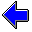Java Security Settings:
This web page employs Java, which requires specific security settings for correct operation.
If the applets on this page do not run correctly, consult the Virtual Chemistry Experiments FAQ
or the Physlet Physics web site for establishing the correct security settings.

# Kinetic Molecular Theory

## Diffusion

In a sample of air at room temperature, the nitrogen molecules, which make up nearly 80% of the air, are moving at a speed of 421 m/sec or 941 mph.

One would therefore expect that a nitrogen molecule on the far side of a room would travel to the opposite end of the room (even a very large room) in a small fraction of a second.

Test this prediction using the simulation below. All of the molecules in the simulation are the same, but one molecule has been colored red and will be used as a tracer. The initial position of the red molecule is defined to be x = 0.0 and y = 0.0; as the simulation runs, the red molecule will move away from its initial position. As the simulation progresses, the displacement of the red molecule has traveled from its initial position will be recorded and displayed on the graph (red line).

The displacement expected for the red molecule if it travels the predicted speed of 421 m/sec is shown by the blue line on the graph.

Are the two lines the same (within the precision of the simulation)? Does the red molecule travel further than expected? Does it travel a shorter distance than expected?

Closely examine the behavior of the molecules in the simulation. Explain why the red line on the graph differs from the blue line. Ultimately, of course, the displacement of the red molecule is limited by the size of the simulation box. The red line deviates from the blue line long before the red molecule reaches one of the walls.

The motion of the red molecule in this simulation is described as diffusion.

### Random Walk

The motion of a particle undergoing diffusion is sometimes described as a random walk.

Run the simulation shown below and observe the path of the red molecule as plotted on the graph at the lower right. The graph shows the x-y position of the red molecule as it moves through the system.

Does the red molecule follow straight-line motion?Pressure-Temperature RelationKinetic Molecular Theory Home PageVirtual Chemistry Home Page

Diffusion.html version 1.2
© 2001-2014 David N. Blauch